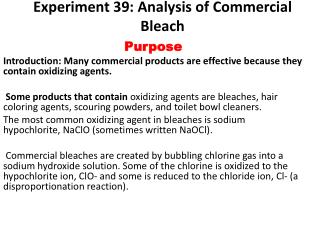DownloadDownload PresentationExperiment 39: Analysis of Commercial Bleach

# Experiment 39: Analysis of Commercial Bleach

Download Presentation## Experiment 39: Analysis of Commercial Bleach

- - - - - - - - - - - - - - - - - - - - - - - - - - - E N D - - - - - - - - - - - - - - - - - - - - - - - - - - -
##### Presentation Transcript

1. Experiment 39: Analysis of Commercial Bleach Purpose Introduction: Many commercial products are effective because they contain oxidizing agents. Some products that contain oxidizing agents are bleaches, hair coloring agents, scouring powders, and toilet bowl cleaners. The most common oxidizing agent in bleaches is sodium hypochlorite, NaClO (sometimes written NaOCl). Commercial bleaches are created by bubbling chlorine gas into a sodium hydroxide solution. Some of the chlorine is oxidized to the hypochlorite ion, ClO- and some is reduced to the chloride ion, Cl- (a disproportionation reaction).

2. The chemical equation for the process is: • Cl2(g) + 2OH- (aq) ---> ClO- (aq) + Cl- (aq) + H2O Terms Oxidizing agent – transfers oxygen atoms or gains electrons Oxidized – to give electrons Reduced- compound gains electrons

3. The amount of hypochlorite ion (ClO- ) present in a solution of bleach can be determined by oxidation-reduction titration. • One of the best methods is the iodine-thiosulfate titration procedure. Iodide ion, I-, is easily oxidized by almost any oxidizing agent • (It has many electrons to lose!). In an acid solution, hypochlorite ions oxidize iodide ions to form iodine, I2. • The iodine that forms is then titrated with a standard solution of sodium thiosulfate.

4. The analysis takes place in a series of steps: • 1.Acidified iodide ion is added to hypochlorite ion solution, and the iodide is oxidized to iodine. 2H+ (aq) + HOCl(aq) + 2I-(aq) ---> Cl-(aq) + I2(aq) + H2O (l) reduced Oxidizing agent oxidized

5. 3.The Iodine solution is titrated with a standard solution of thiosulfate ions, which reduces the iodine back to iodide ions: • I2+ (2S2O3) 2----> 2I-+ (S4O6 )2-

6. Part ADilute 1M Na2S2O3 to 0.05M Na2S2O3 • Pour 5 ml of 1M Na2S2O3 into a 250 ml beaker • Dilute with DI water until it reaches 100 ml • This will create a 0.05M Na2S2O3

7. Part A standardization of Na2S2O3 • Rinse and fill a buret with Na2S2O3 • Rinse and fill a second buret with .01 M KIO3 • Dispense 15 ml of KIO3 solution into a 250 ml Erlenmeyer flask • Add to flask 25 ml of water • 3ml of 3M KI • 2ml of 3M H2SO4

8. Part A begin titration • Slowly add Na2S2O3 • When the solution turns a light yellow add 0.5ml of starch indicator, the solution will turn black • Continue to add Na2S2O3 until the solution reaches an endpoint (turns colorless) • Calculate Molarity of Na2S2O3 using the formula below as seen on page 481 lO3-2 + 6S2O3-2 + 6H+ ---> l- + 3S4O6- 2+ 3H2O

9. Calculations for standarization • Moles of lO3-2 = (.01 MlO3-2 x volume of lO3-2) • 6 x Moles of lO3-2 = moles of Na2S2O3

10. Part B Determination of the oxidizing agent of bleach • Fill buret with 0.05M Na2S2O3 • Add 0.5g of bleach into a 250ml flask • Record the mass of the unknown bleach

11. Part B Determination of the oxidizing agent of bleach • To the flask add • 25 ml of di water • 3ml of 3M KI • 2ml of 3 M H2SO4 • 5 drops of 3 % ammonium molybdate It should turn a dark brown, if it does not call me over If it does immediately begin to titrate 2H+ (aq) + HOCl(aq) + 2I-(aq) ---> Cl-(aq) + I2(aq) + H2O (l)

12. Part B begin titration • Slowly add Na2S2O3 to flask • When the solution turns a light yellow add 0.5ml of starch, the solution will turn black\ • Continue to add Na2S2O3 until it turns clear I2+ (2S2O3) 2----> 2I-+ (S4O6 )2-

13. Part B begin titration • Calculate Moles of NaOClusing the formula below as seen on page 480 NaOCl + 2S2O3-2 + H+ ---> Cl- + S4O6+ H2O

14. Calculations for titration • Moles of Na2S2O3= (.05 MNa2S2O3x volume of Na2S2O3) • 1/2moles of Na2S2O3 = Moles of NaOCL

15. Calculations for % oxidizing agent (OCL) • Mass NaOCl = Moles of NaOCl x (74.5g/mol NaOCl ) Mass of NaOCl Mass of unknown bleach X 100 = % oxidizing agent